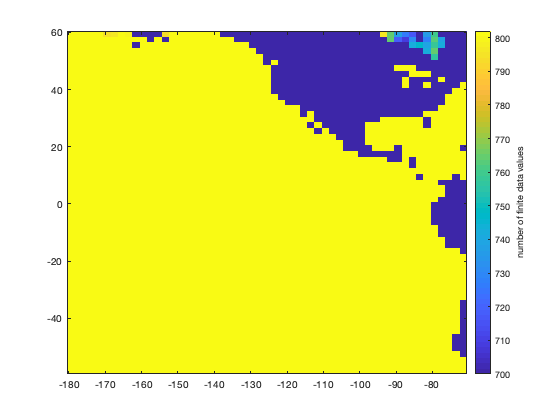# detrend3 documentation

detrend3 performs linear least squares detrending along the third dimension of a matrix.

## Syntax

```Ad = detrend3(A)

## Description

Ad = detrend3(A) removes the linear trend from the third dimension of A assuming slices of A are sampled at constant intervals.

Ad = detrend3(A,t) specifies times t associated with each slice of A. Times t do not need to occur at regular intervals in time.

Ad = detrend3(...,'omitnan') applies detrending even in grid cells that contain NaN values. If many grid cells contains spurious NaNs, you may find that this option is slower than the default.

## Example 1: A 3D gridded dataset

This sample dataset has a trend of 3.2 units/timestep everywhere:

```t = 50:50:1000;
y = 3.2*t + 0.1*randn(size(t)); % the trend is 3.2*t (plus some random noise)

% Create 5x5 grid w/trend dictated by y:
Z = expand3(ones(5),y);
```

Here's proof via the trend trend function:

```trend(Z,t)
```
```ans =
3.2000    3.2000    3.2000    3.2000    3.2000
3.2000    3.2000    3.2000    3.2000    3.2000
3.2000    3.2000    3.2000    3.2000    3.2000
3.2000    3.2000    3.2000    3.2000    3.2000
3.2000    3.2000    3.2000    3.2000    3.2000
```

Now let's detrend:

```Z_detrend = detrend3(Z,t);
```

And now what's the trend?

```trend(Z_detrend,t)
```
```ans =
1.0e-14 *
-0.2212   -0.2212   -0.2212   -0.2212   -0.2212
-0.2212   -0.2212   -0.2212   -0.2212   -0.2212
-0.2212   -0.2212   -0.2212   -0.2212   -0.2212
-0.2212   -0.2212   -0.2212   -0.2212   -0.2212
-0.2212   -0.2212   -0.2212   -0.2212   -0.2212
```

Just numerical noise (note that it is zero to the level of 1.0e-14).

## Example 2: Sea Surface Temperatures

This example calculates an area-averaged sea surface temperature anomaly from a gridded time series, then detrends the gridded sst time series and plots the detrended anomaly time series.

Begin by loading the sample pacific_sst.mat dataset of monthly SSTs. Use cdtarea to get the area of each grid cell, and then use local to get the 1D time series of area-weighted ssts for all ocean grid cells.

```% Load example data:

% Turn lat,lon arrays into grids:
[Lon,Lat] = meshgrid(lon,lat);

% Get the area (m^2) of each grid cell:
A = cdtarea(Lat,Lon); % area of each grid cell (m^2)

% Make a mask of only finite data (to effectively ignore land)

% Get 1D time series of area-weighted sst:
```

Now plot the area-weighted sst anomaly using the anomaly function. For context, plot the linear trend with polyplot:

```figure
anomaly(t,sst_1-mean(sst_1))
axis tight
datetick('x','keeplimits')
ntitle('sea surface temperature anomaly (not detrended)')

hold on
polyplot(t,sst_1-mean(sst_1),1,'k','linewidth',3)
```Now detrend the entire 3D sst dataset and plot the same area-averaged time series, but this time using the detrended data:

```% Detrend the full 3d time series:
sst_dt = detrend3(sst);

% Get the area-averaged detrended SST time series:

figure
anomaly(t,sst_dt_1-mean(sst_dt_1))
axis tight
datetick('x','keeplimits')
ntitle('DETRENDED sea surface temperature anomaly')

hold on
polyplot(t,sst_dt_1-mean(sst_dt_1),1,'k','linewidth',3)
```## Example 3: The 'omitnan' option

In some cases, your gridded dataset may have spurious NaNs in them. That is the case for the sst dataset we analyzed in the example above. See, for the 802 months of SST data, look at how many values are finite:

```figure
imagescn(lon,lat,sum(isfinite(sst),3))
cb = colorbar;
ylabel(cb,'number of finite data values')
caxis([700 802]) % sets color axis limits
```In the figure above, take a look at Hudson Bay in Canada. You'll notice that not all of the 802 months have valid data there. As many as 100 months of data are missing because it can be hard to get a good SST reading in the winter months when ice screws up the measurement. Still, you may need to remove the long term trend from the available data.

Here's a comparison of detrending the sst dataset without versus with the 'omitnan' option. Start by using the trend function, simply to assess the magnitude of the trend:

```figure
subplot(1,2,1)
imagescn(lon,lat,trend(sst,12))
cb = colorbar('location','southoutside');
xlabel(cb,'SST trend \circC/yr')
title 'trend without omitnan'
caxis([-0.04 0.04])
cmocean('balance')

subplot(1,2,2)
imagescn(lon,lat,trend(sst,12,'omitnan'))
cb = colorbar('location','southoutside');
xlabel(cb,'SST trend \circC/yr')
title 'trend with omitnan'
caxis([-0.04 0.04])
cmocean('balance')
```In the figure above, note the difference in Hudson Bay, Canada.

Now detrending works the same way. We'll detrend each dataset, and then plot the trends of the detrended datasets just as above:

```sst_dt = detrend3(sst);
sst_dt_o = detrend3(sst,'omitnan');

figure
subplot(1,2,1)
imagescn(lon,lat,trend(sst_dt,12))
cb = colorbar('location','southoutside');
xlabel(cb,'SST trend \circC/yr')
caxis([-0.000000000000001 0.000000000000001])
cmocean('balance')
title 'detrended trend without omitnan'

subplot(1,2,2)
imagescn(lon,lat,trend(sst_dt_o,12,'omitnan'))
cb = colorbar('location','southoutside');
xlabel(cb,'SST trend \circC/yr')
caxis([-0.000000000000001 0.000000000000001])
cmocean('balance')
title 'detrended trend with omitnan'
```Now the only "trend" that remains in the detrended dataset is numerical noise (Note the 1x10^-15 limits of the color scale.)

## Author Info

This function is part of the Climate Data Toolbox for Matlab. The function and supporting documentation were written by Chad A. Greene.